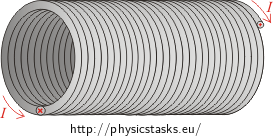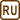## Varying Magnetic Flux trough Solenoid

Determine how many turns a solenoid must have, in order to induce a voltage of 30 V provided that the magnetic flux in its cavity changes from 50 mWb to 20 mWb per time 0.10 s.• #### Hint

Evaluate the number of turns of the solenoid from Faraday's law of electromagnetic induction, which states that the size of electromotive voltage induced in the solenoid is proportional to the change in magnetic flux through the solenoid per given time. The induced voltage is also proportional to the number of turns of the solenoid.

• #### Analysis

The electromotive force induced in one turn of the solenoid is equal to the rate of change of magnetic flux. If we are interested in the size of the induced voltage in the whole solenoid, we must multiply the rate of the change of magnetic flux by the number of turns.

The change in magnetic flux per time is determined as the difference between two values of the magnetic flux.
• #### Solution

We determine the number of turns of the solenoid from Faraday's law of electromagnetic induction. Mathematical notation of this relationship is

$|U_i|=N|\frac{\mathrm{\Delta}\Phi}{\mathrm{\Delta} t}|,\tag{1}$

where ΔΦ is the difference of magnetic flux

$|\mathrm{\Delta}\Phi |= |\Phi_2 - \Phi_1|.$

The expression is substituted into the equation (1)

$|U_i|=N\frac{|\Phi_2 - \Phi_1|}{\mathrm{\Delta}t}.$

We evaluate the number of turns N

$N=\frac{|U_i|}{|\Phi_2 - \Phi_1|}\,\mathrm{\Delta}t.$

Note: We use the absolute value for the voltage and the magnetic flux difference, because we are only interested in their sizes.

• #### Given values and numerical substitution

$U_i=30\,\mathrm{V}$ $\Phi_1=\,50\,\mathrm{mWb}=\,50{\cdot}10^{-3}\,\mathrm{Wb}$ $\Phi_2=\,20\,\mathrm{mWb}=\,20{\cdot}10^{-3}\,\mathrm{Wb}$ $\mathrm{\Delta}t=\,0.10\,\mathrm{s}$ $N=\,?$
$N=\frac{|U_i|}{|\Phi_2 - \Phi_1|}\,\mathrm{\Delta}t=\frac{30}{|20{\cdot}10^{-3} - 50{\cdot}10^{-3}|}\cdot0.10=100$